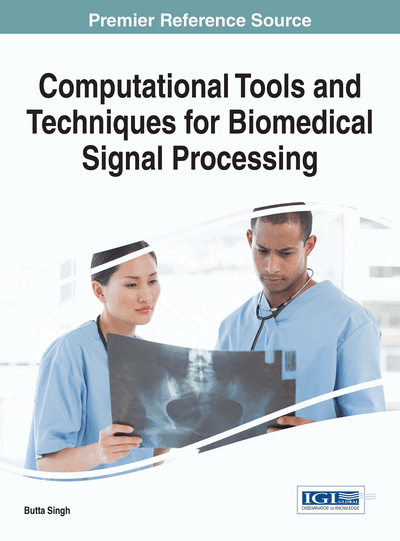# Parameter Estimation of Nonlinear Biomedical Systems Using Extended Kalman Filter Algorithm: Development of Patient Specific Models

Kamalanand Krishnamurthy (Anna University, MIT Campus, India)
DOI: 10.4018/978-1-5225-0660-7.ch004

## Abstract

Parameter estimation is a central issue in mathematical modelling of biomedical systems and for the development of patient specific models. The technique of estimating parameters helps in obtaining diagnostic information from computational models of biological systems. However, in most of the biomedical systems, the estimation of model parameters is a challenging task due to the nonlinearity of mathematical models. In this chapter, the method of estimation of nonlinear model parameters from measurements of state variables, using the extended Kalman filter, is extensively explained using an example of the three-dimensional model of the HIV/AIDS system.
Chapter Preview
Top

## Introduction

Mathematical modeling of complex biomedical systems is highly useful for analysis of the actual system under investigation. Mathematical modeling can improve our understanding of several diseases and is highly useful for diagnosis and prognosis of several diseases. In the context of modeling, doctors and engineers can work together to identify diseased states, for development of diagnostic tools and for devising proper treatment strategies (Kamalanand & Ramakrishnan, 2015).

In biology, mathematical models are often developed to mimic the physiological systems. For example, mathematical models of organs of the body such as the heart, lungs, pancreas etc, are highly useful for understanding and analyzing the normal and abnormal functionality of the organ under investigation (Kamalanand & Srinivasan, 2011). Another class of biomedical models involves the modelling of cell growth and population growth of certain pathogens. These models are highly useful for analyzing the growth of cancer cells and for identification of suitable drugs for inhibiting harmful cell growth. Further, a class of models namely the predator-prey models has played an important role in modelling of biological processes. For example, the modelling of the interaction of certain pathogens with the human immune system and the modelling of co-infections (two of more infections simultaneously affecting an individual) fall under the class of predator-prey models. Such models have been proved to be highly useful for diagnosis of several infectious diseases (Mannar Jawahar & Kamalanand, 2013). Also, in recent years, the growth of computational models in the field of neurobiology has led to the improved understanding of brain functions and in development of systems based on artificial intelligence. For example, the models of biological processes developed using artificial intelligence approaches such as the artificial neural networks and support vector machines have several applications in diagnostics and pattern detection (Manickavasagam, Sutha, & Kamalanand, 2014).

The generation of a suitable mathematical model based on ordinary differential equations (ODE) or partial differential equations (PDE) is also useful for predicting the future states of the system and for analysis of the system as a whole (Filter, Xia, & Gray, 2005; Lillacci & Khammash, 2010; Arunachalam, Jacob, & Kamalanand, 2014). The development of such a model involves identification of several complex interrelationships in the system and is a time consuming task. After development of such a model which describes the actual biological system, the model must be tuned to specific cases. In other words, a mathematical model has to be patient specific so that it can be properly used for diagnosis and treatment planning applications. Hence, the important task is to tailor the model to match the measured variables of the individual patient under study (Ho & Chan, 2010, Kamalanand & Jawahar, 2015).

The patient specific mathematical models are highly useful for:

• 1.

Predicting the progression of the disease for individual patients.

• 2.

Predicting the health of the individual.

• 3.

Surgery planning for a specific patient.

• 4.

Therapy planning for a specific patient.

The process of developing a patient specific model involves estimation of the model parameters from the measured state variables of the patient. In the case of parameter estimation of ODE models, the estimation problem is considered as a linear estimation case when the model is a linear model and a nonlinear estimation problem when the model is nonlinear. Further, the estimation problem can be classified into online and offline estimation. In offline estimation, the model parameters are estimated after the state variables are measured for the specific time interval. However, in online estimation, the model parameters are updated for each and every sampling instant.

## Complete Chapter List

Search this Book:
Reset